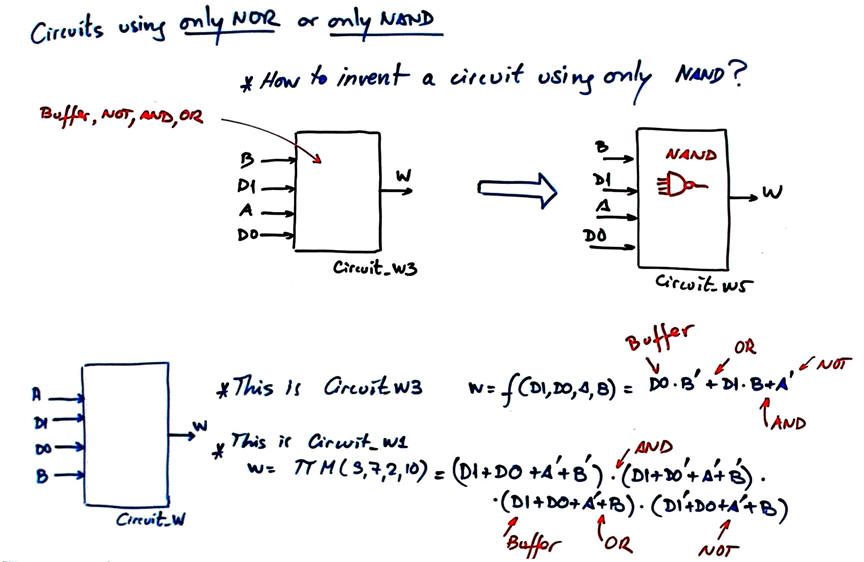﻿ Digital Circuits and Systems - Circuits i Sistemes Digitals (CSD) - EETAC - UPCBachelor's Degree in Telecommunications Systems and in Network EngineeringLecture 5 L1.5: Designing circuits using only-NOR and only-NAND P1 design plan A: designing circuits using only one kind of gates [24/2]

1.5.2.1.3. Only-NOR gates

1.5.2.1.4. Only-NAND gatesFig. 1. Examples of circuits designed from the initial specifications (truth table). Equations can be modified to invent circuits using only one type of gate: NOR or NAND.

Now that you know how to use the minimiser minilog, what is left to finish discussion on P1 design plans is to examine how to build circuits from the same truth table using only-NAND (Circuit_5, Circuit_7, Circuit_10) or only-NOR gates (Circuit_6, Circuit_8).Fig. 2. Class discussion on how to create Circuit_W5 from Circuit_W3. Step1: learn to build any gate using only-NOR or only-NAND. Step 2: learn how to convert a complete equation. Step 3: complete your classnotes and apply these concepts to create other circuits, for instance: how to build Circuit_W11 from Circuit_W3 using only 2-input NOR gates? how to build Circuit_W12 from Circuit_W8 using only 2-input NOR gates?

Study also these notes: circuits using only NAND or only NOR gates. This is a trick to use only-NOR2 or only-NAND2

This Circuit_K3 is another complete design example.

1.5.2.1.5. Any kind of nonstandard equation.

Thus, at this point you see how the many example circuits presented in P1 are conceived mixing any combination of logic equations. CSD course is totally based on examples and tutorials the link to which is always below specifications of highlighted projects.